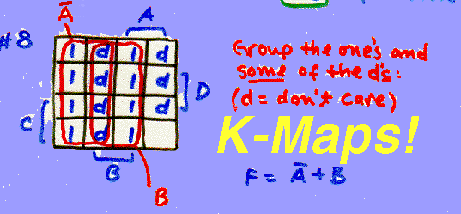This quiz is designed to give you practice in simplifing Boolean expressions through the use of K-Maps. Read the appropriate sections in the text and answer the questions below. (Go back to homepage.)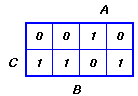Identify the function which generates the K-map shown ``` ```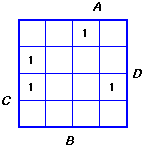Identify the function which generates the K-map shown ``` ```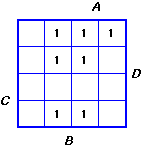Identify the most simple SOP expression from the K-map shown. ``` ```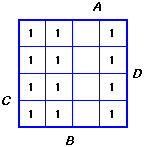Identify the most simple SOP expression from the K-map shown. ``` ```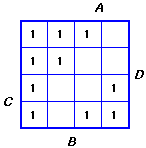Identify the most simple SOP expression which generates the Karnaugh map shown: ``` ```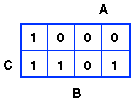Identify the most simple POS expression which generates the K-map shown. ``` ```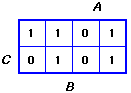Identify the most simple POS expression which generates the K-map shown. ``` ```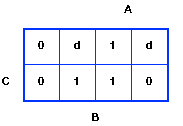The most simple expression which can be obtained from the K-map shown is: ``` ```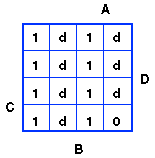The most simple SOP expression which can be obtained from the K-map illustrated is: ``` ```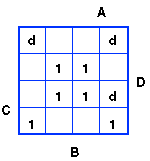The most reduced expression which can be obtained from the K-map illustrated is: ``` ```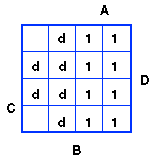The most reduced expression which can be obtained from the K-map illustrated is: ``` ```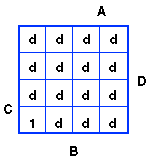The most simple expression which can be obtained from the K-map illustrated is: